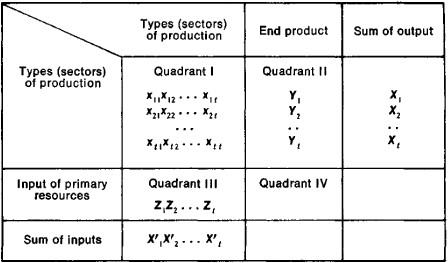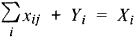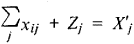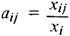# Matrix Models

The following article is from The Great Soviet Encyclopedia (1979). It might be outdated or ideologically biased.

## Matrix Models

in economics, one of the most common types of mathematical economic models. The components of these rectangular tables reflect the interrelationships of economic elements; the tables have a defined economic meaning, the values of which are computed by rules established in matrix theory. Matrix models reflect the structure of production input and the distribution of output and newly created value.

Matrix models are standardized balance models; they bring together in a single table the balances of output distribution (by specific types), the related balances for inputs to produce the output, and the rates for material and monetary inputs. Matrix models are used for economic analysis and plan calculations employing computer technology.Figure 1. The fundamental diagram of a matrix model

The matrix model of an economic unit shown in Figure 1 has the shape of a rectangular table divided into four quarters (quadrants). In the matrixthe elements of line xij are deliveries of output from subdivision (sector) i to subdivision (sector) j, Yi, is the final output of subdivision (sector) i, and Xi is the gross output of subdivision (sector) i. The equations of the lines of the matrix are balances of the distribution of output produced in different production subdivisions (for example, in the shops of an enterprise), in different economic units (enterprises, associations), and in different sectors of the national economy. Their economic meaning is perfectly clear: the deliveries within the production system and the final product constitute the gross output of the subdivision (sector). Equally obvious is the meaning of the equation composed of the elements of the matrix columns:Here, xij represents inputs of the output of subdivision (sector) j to produce the output of subdivision (sector) i, Zj is inputs of primary resources and newly created value in subdivision (sector) j and X′j is gross expenditures together with newly created value in subdivision (sector) j.

Xi = X′j, if i is the same as j. Then the law of value finds expression in this equality of the sums of similar lines and columns: the value of goods and services distributed and accumulated is equal to the value of production expenditures plus newly created value. A whole series of other derivative equations follows from this basic equality of the matrix model; these equations make such models a convenient tool for planning and analytic calculations.

Thus, each index has a dual meaning. On the one hand it expresses the volume of deliveries from one production subdivision (sector) to another, whereas on the other it reflects the volume of product consumption by the second subdivision of the output of the first. Therefore, the first quadrant (I) of a matrix model reflects the internal production links of the economic system being modeled. The production structure receives its clearest quantitative expression in coefficients of direct expenditures, which are the quotients of dividing the expenditures of the output of all subdivisions by the output of a particular subdivision:Then the first quadrant of the matrix model becomes a table of standardized inputs, calculated per unit of gross output of each type of output. As a result of converting the inverted quadratic matrix, the first quadrant receives coefficients of total expenditures that express the aggregate of direct and indirect expenditures calculated per unit of final output B = (E − A)−1. The results of production and economic activity (final output) are reflected in quadrant II; this is considered the output of the model. Quadrant III reflects inputs of primary resources received in the system from outside and newly created value (embodied labor); this is considered the input of the model. In quadrant IV, at the point where the lines of the third quadrant intersect the columns of the fourth quadrant, the transit processes of passing along material resources and redistributing value are reflected: the resources arriving as input for the given economic system are used as final products at the output stage, bypassing the production subdivisions.

Because of its simplicity of form and rich economic meaning, the matrix model is used extensively in various areas of the economy for planning and for making statistical calculations, organizing the system of rates and standards, standardizing documentation and reducing document circulation, organizing economic accountability within production units, and doing economic analysis.

Matrix models designed for internal plant planning and for production records are very large-scale tables (up to several hundred positions) that include production rates for inputs of raw materials, processed materials, assembly components, and machine and worker time to produce each particular type of output and the assemblies and parts that go into it. The properties of matrix multiplication are used for simultaneous representation of the production-technological structure and the organizational structure. A characteristic of matrix models is that the plan or analytical calculation is done in one procedure for the entire production and economic system; as a result there is complete uniformity and mutual coordination among all divisions of the plan (or report), including production, supply, finance, labor and wages, and prime cost. This unified procedure also makes it possible to correct various types of rates on a standing basis and to coordinate them with one another. If matrices become too large and calculations are done by computer, tables are not ordinarily constructed but rather the appropriate data are put on punched cards or magnetic tape; the matrix serves simply as a calculation scheme.

The matrix-type technical, industrial, and financial plan (tekhpromfinplan) of an enterprise is a series of standardized documents; the chief one is a matrix model of the enterprise’s economy (in consolidated indexes as compared to “technological” matrices). The summary balance of the enterprise’s economy is decoded in a series of tables of detailed indexes for materials supply, labor, fixed assets and equipment, and enterprise finances, which have a uniform matrix form. The matrix-type technical, industrial, and financial plan is a very refined form of standardized documentation adapted for machine processing. In the matrix-type plan the number of indexes and tabular forms is much decreased, although the same amount of data is retained; moreover, all indexes are given in comparable and mutually coordinated form.

Matrix models are also used for the economies of national economic sectors, republics, and territorial production complexes as well as for the country’s national economy. Matrices of this type are called intersectorial balances (input-output tables) and are used extensively in planning and statistics.

Matrix models that are used to model successive links of the national economy on the basis of the rules of matrix addition form a single, mutually interrelated complex, which is called a system of matrix models. Thus, the matrix model of the economy of a sector is created by joining together the matrix models of the enterprises, using what are called variant matrices, which reflect different technological variants for producing goods and services at different enterprises. These variant matrices are important in their own right for interplant and intersectorial analysis and for the establishment of rates and standards for the sector. Subtracting and dividing the matrices takes care of the process of allotting the sectorial plan by enterprises, whereas their representation in the form of systems of linear equations is ensured by the use of mathematical programming methods for optimal sectorial planning. Intersectorial balances of republic economies and the national economy as a whole can be constructed on the basis of consolidated sectorial matrices.

The matrix models system serves as the basis for projecting integrated schemes for processing economic data in the automated control systems of departments and ministries, enterprises, and planning and statistical bodies. The process of integrated data processing itself is reflected in the information matrix model. In this case, xij no longer signifies mutual deliveries of goods and services but rather represents the transmission of definite messages evaluated in certain information units (documents, indexes, bits of information). Matrices are also used to model transportation flows, the processes of population migration and movement of labor resources, the work of organizational structures, the processes of decision-making, and any other processes for which the balance equation is effective.

The matrix model is convenient for analysis because it gives a simple and graphic representation of the characteristics of a highly diverse range of objects wherever there is a balance of receipts and expenditures of physical assets, energy, values, information, and the like and where the relationships are direct and linear. Matrix analysis provides a number of new possibilities compared to other methods of economic analysis: interpolation of unobserved elements, identification of the logical structure of production and economic processes, detailed consideration of the mutual influence of factors, and employment of the methods of mathematical programming for analyzing plan optimality. Matrix analysis is used to study the economic activity of enterprises, production associations, sectors, regional economies, republic economies, and the country’s national economy. Matrix analysis is also applied to the processes of economic management (analysis of document circulation, movement of indexes, interrelations of management tasks) and to distinct economic processes (including the bookkeeping balance and the movement of cash money).

The characteristics of block matrices permit the graphic representation of complex interrelationships and establish the matrix as a convenient tool for logical analysis of complex structures where the technological, production organization, and economic aspects of the activity of national economic sectors are reflected simultaneously. Thus, matrix models of the production process at an enterprise are used to identify production “loops” and irrational links and to investigate equipment load and the use of work force. The “information matrix,” which reflects the movement of documents and indexes, is used to analyze the rationality of the structure, labor organization, and workloads of divisions of the plant administration, the organization, and the ministry. Economic matrix models, that is, models of economic units constructed in comparable value indexes, are used to analyze the interaction of different types of activity at a particular site; these activities, taken as a whole, constitute the economic activity of the enterprise, production association, or sector.

Matrix models of an analogous type but constructed for larger economic systems such as economic regions and republics are used to analyze the balance and proportionality of the plan and the degree of utilization of particular types of resources (production capacities and labor, materials, and financial resources) and to analyze and plan the amalgamation and specialization of production.

For dynamic analysis, series of matrix models are compared for successive periods of time, or there is a paired comparison of plan and report models.

Matrix models of republic economies, regional economies, and the country’s national economy (intersectorial balances) are used for an expanded system of analytic calculations. These models are used to identify the principal national economic proportions, the ratio between material production and the nonproduction sphere, and the ratios among basic cost elements. They are also used to show the shares of sectors, regions, and republics in the creation of the country’s national income and aggregate social product. In addition, projected plans for the construction of new enterprises in republics and regions, the incorporation of new agricultural land, and the construction of new cities are analyzed on the basis of territorial matrix models. The comprehensive efficiency of these activities for the country, republic, or region as a whole is considered at this point; personal incomes are computed, and the structure of public demand is brought to light.

Using the methods of electronic simulation, matrix models make it possible to investigate flows of physical assets, services, and finances in their development. A system of matrix models makes it possible to “play out” projected construction plans in order to analyze the economic consequences of carrying out plan variations that are difficult to compare directly. Information flows created in economic management agencies when the national economic plan is being drawn up and its implementation is being monitored can also be analyzed using matrix models.

### REFERENCES

Nemchinov, V. S. Ekonomiko-matematicheskie metody i modeli. Moscow, 1962.
Cherniak, Iu. I. Mezhotraslevoi balans i ego ispol’zovanie v ekonomicheskom analize i planovykh raschetakh, issue 1. Moscow, 1962.
Cherniak, Iu. I. “Edinstvo planirovaniia proizvodstva, snabzheniia i finansirovaniia v sisteme matrichnykh modelei.” In the collection Primenenie matematiki v ekonomicheskikh issledovaniiakh, vol. 3. Moscow, 1965.
Voloshin, N. I. “Sistema matrichnykh modelei vn trizavodskogo planirovaniia.” Ibid. Moscow, 1965.
Makhrov, N. V. “Metod mezhotraslevogo balansa—osnova svoda nizovykh planov.” Ibid. Moscow, 1965.
Budris, V. A. “Obrabotka delovoi informatsii, predstavlennoi v matrichnoi forme.” In the collection Matematiko-ekonomicheskie problemy: Trudy mzhvuzovskoi nauchnoi konferentsii. [Leningrad] 1966.
Ershov, E. B. “O vyiavlenii i ispol’zovanii strukturnykh osobennostei matrits v zadachakh planirovaniia.” Ekonomika i matematicheskie metody, 1966, vol. 2, issue 2.

IU. I. CHERNIAK

Mentioned in ?
References in periodicals archive ?
Since the boundary of the positive cone is always invariant for semelparous Leslie matrix models of any dimension, see , initial conditions of the form (v,0) or (0, w) in the 2-dimensional case will always produce SYC dynamics.
Caption: Figure 4: The power output using prototype and 5-order matrix models in primary frequency regulation mode after frequency disturbance.
Another advantage of this approach is that it is able to establish rock matrix models , which can be assembled with the corresponding pore model and used for the multiphysics coupled simulation.
In the discrete case, such matrix models (often referred to as Leslie matrix models) were independently developed in the 1940s (see [6-9]), but perhaps somewhat strange not adapted by ecologists until the 1970s (cf.
Matrix models of long-lived species such as abalone are not normally considered sensitive to the reproductive terms (Crouse et al.
At just 35 inches long and 30 'A inches wide, the Bulldog is more maneuverable than previous Matrix models and its Recoil Energy Dissipation System quells shot noise and vibration.
Two growth models in particular, however, hold promise for characterizing the achievement growth on alternate assessments: transition matrix models and multilevel linear growth models.
Outlining a connection from random matrices to the six-vertex model of statistical physics, Bleher and Liechty focus on the Riemann-Hilbert method for both continuous and discrete orthogonal polynomials, and applications of this approach to matrix models as well as to the six-vertex model.
The element quality of both pore space and rock matrix models is shown in Figure 4.
Tables of examples, charts showing operational resource flow and matrix models, and more provide clear, specific details, discussions and exam prototypes.
Federal regulators have been investigating the possibility of stalling engines in the Corolla and Matrix models since December and intensified their probe earlier this week.

Site: Follow: Share:
Open / Close